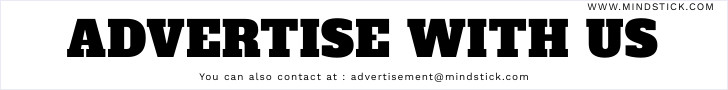# find the length of array?

Posted by  Somesh
4916  View(s)
Ratings:
Rate this:
how i can find the length of Array in VB script?
Give the soln of its
1.##### Re: find the length of array?

Hi.....

Use following code snippet to calculate length of array in vbscript

Dim NewArray(10)
NewArray(0) = "Apple"
MsgBox arrLength(NewArray)

Function arrLength(vArray)

ItemCount = 0

For ItemIndex = 0 To UBound(vArray)
If Not(vArray(ItemIndex)) = Empty Then
ItemCount = ItemCount + 1
End If
Next
arrLength
= ItemCount
End Function